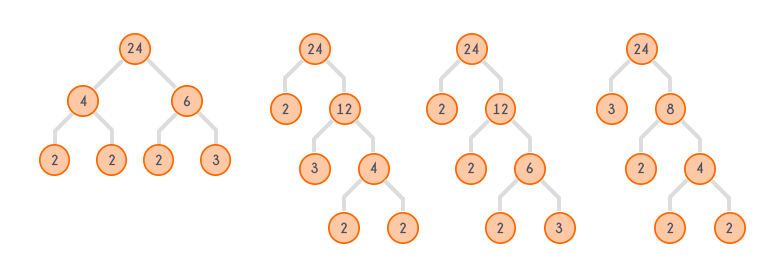Factor Trees
/

## Dynamic Programming, Factorization, Mathematics, Recursion, Sieve

Problem
Editorial
Analytics

A factor tree is a binary tree where each non-leaf node p has two children q and r, such that $p = q * r$ and $r \ne 1, q \ne 1$. Prime factors are treated as leaf nodes of the tree.
Following are the factor trees of $24$ :These are the four possible factor trees of $24$.
Since Fredo loves doing experiment, he wants to know how many different factor trees are there whose root lies in the range $[l,r]$ and the tree contains x as a node.

Two factor trees are different if and only if they are not isomorphic.

Two trees are called isomorphic if one of them can be obtained from other by a series of flips, i.e. by swapping left and right children of a number of nodes. Any number of nodes at any level can have their children swapped. Two empty trees are isomorphic.These two trees are isomorphic.

The above four factor trees of $24$ are different as they hold the above definition.

Fredo has written a program to know the different factor trees as asked in the question but he asks you to write a program to check whether he did it right or not.

Input:
First line consists of an integer T, representing the number of test cases.
Each of the following T lines consists of three integers $x,l,r$ as described in the question.

Output:
Print the number of different factor trees for each query in a separate line.

Constraints:

• $1 \le T \le 10^5$
• $2 \le x \le 5 * 10^2$
• $2 \le l \le r \le 5 * 10^3$
SAMPLE INPUT
2
6 2 24
16 192 192

SAMPLE OUTPUT
5
18

Explanation

Test case 1:
6 is present in factor trees of $6,12,18$ and $24$.
There is one factor tree of 6 containing 6, one factor tree of $12$ containing 6, one factor tree of $18$ containing 6 as a node.
$24$ has two different trees containing 6. One tree has 2 and $12$ as factors of $24$, other tree has 4 and 6 as factors of $24$.
In all, 6 comes in 5 different factor trees in the range $[2,24]$.

Time Limit: 1,0 sec(s) for each input file.
Memory Limit: 256 MB
Source Limit: 1024 KB

## This Problem was Asked in

Initializing Code Editor...
Уведомления

?